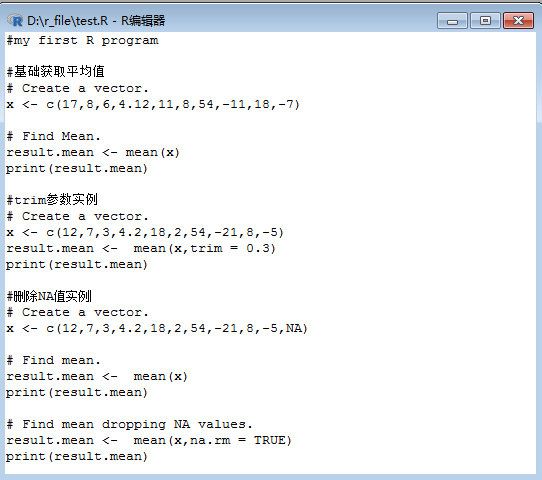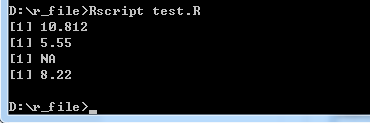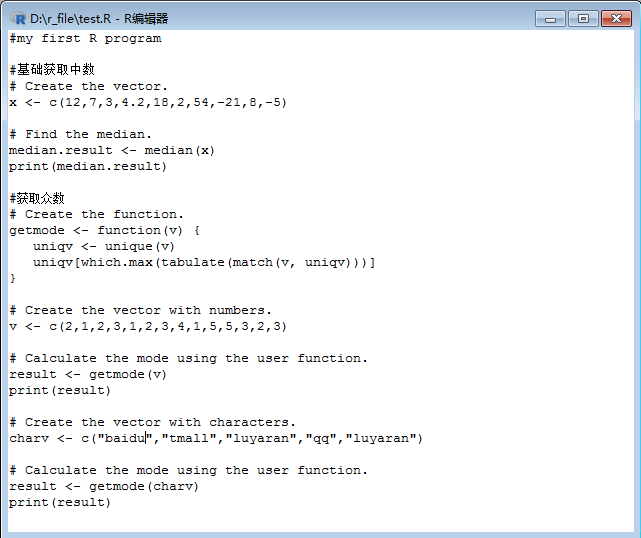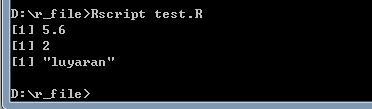• > a=c(70,80,60) > mean(a) #平均值  70 > wt=c(3,3,4) > weighted.mean(a,wt) #加权平均值  69 转载于:https://www.cnblogs.com/jmlovepython/p/6909703.html


> a=c(70,80,60)
> mean(a)  #平均值
 70
> wt=c(3,3,4)
> weighted.mean(a,wt) #加权平均值
 69

转载于:https://www.cnblogs.com/jmlovepython/p/6909703.html
展开全文• R语言平均值，中位数和众数 R中的统计分析通过使用许多内置函数来执行的。这些函数大部分是R基础包的一部分。这些函数将R向量与参数一起作为输入，并在执行计算后给出结果。 我们在本章中讨论的是如何求平均值，...

R语言平均值，中位数和众数
R中的统计分析通过使用许多内置函数来执行的。这些函数大部分是R基础包的一部分。这些函数将R向量与参数一起作为输入，并在执行计算后给出结果。我们在本章中讨论的是如何求平均值，中位数和众数。下面将分别一个个演示和讲解 -
1.平均值
平均值是通过取数值的总和并除以数据序列中的值的数量来计算。函数mean()用于在R中计算平均值。
语法
R中计算平均值的基本语法是 - mean(x, trim = 0, na.rm = FALSE, ...) R 以下是使用的参数的描述 -     x - 是输入向量。     trim - 用于从排序的向量的两端删除一些观测值。     na.rm - 用于从输入向量中删除缺少的值。 示例 # Create a vector.  x <- c(17,8,6,4.12,11,8,54,-11,18,-7) # Find Mean. result.mean <- mean(x) print(result.mean) R 当我们执行上述代码时，会产生以下结果 -  10.812 Shell
1.1.应用修剪选项
当提供trim参数时，向量中的值进行排序，然后从计算平均值中删除所需数量的观察值。
例如，当trim = 0.3时，每一端的3个值将从计算中删除以找到均值。
在这种情况下，排序的向量为(-21，-5,2,3,42,7,8,12,18,54)，从用于计算平均值的向量中从左边删除：(-21，-5,2)和从右边删除：(12,18,54)这几个值。 # Create a vector. x <- c(12,7,3,4.2,18,2,54,-21,8,-5) # Find Mean. result.mean <-  mean(x,trim = 0.3) print(result.mean) R
当我们执行上述代码时，会产生以下结果 -  5.55 Shell
1.2.应用NA选项
如果缺少值，则平均函数返回NA。要从计算中删除缺少的值，请使用na.rm = TRUE。 这意味着删除NA值。参考以下示例代码 - # Create a vector.  x <- c(12,7,3,4.2,18,2,54,-21,8,-5,NA) # Find mean. result.mean <-  mean(x) print(result.mean) # Find mean dropping NA values. result.mean <-  mean(x,na.rm = TRUE) print(result.mean) R 当我们执行上述代码时，会产生以下结果 -  NA  8.22 Shell
2.中位数
数据系列中的中间值被称为中位数。R中使用median()函数来计算中位数。
语法
R中计算位数的基本语法是 - median(x, na.rm = FALSE) R
以下是使用的参数的描述 -     x - 是输入向量。     na.rm - 用于从输入向量中删除缺少的值。
示例 # Create the vector. x <- c(12,7,3,4.2,18,2,54,-21,8,-5) # Find the median. median.result <- median(x) print(median.result) R 当我们执行上述代码时，会产生以下结果 -  5.6 Shell
3.众数
众数是指给定的一组数据集合中出现次数最多的值。不同于平均值和中位数，众数可以同时具有数字和字符数据。
R没有标准的内置函数来计算众数。因此，我们将创建一个用户自定义函数来计算R中的数据集的众数。该函数将向量作为输入，并将众数值作为输出。
示例 # Create the function. getmode <- function(v) {    uniqv <- unique(v)    uniqv[which.max(tabulate(match(v, uniqv)))] } # Create the vector with numbers. v <- c(2,1,2,3,1,2,3,4,1,5,5,3,2,3) # Calculate the mode using the user function. result <- getmode(v) print(result) # Create the vector with characters. charv <- c("baidu.com","tmall.com","yiibai.com","qq.com","yiibai.com") # Calculate the mode using the user function. result <- getmode(charv) print(result) R
当我们执行上述代码时，会产生以下结果 -  2  "yiibai.com"

转载于:https://www.cnblogs.com/amengduo/p/9586549.html
展开全文• R中的统计分析通过使用许多内置函数来执行。 这些函数大多数是R基础包的一部分。...函数mean()用于在R语言中计算平均值。 语法 用于计算R中的平均值的基本语法是 - mean(x, trim = 0, na.rm = ...
R中的统计分析通过使用许多内置函数来执行。 这些函数大多数是R基础包的一部分。 这些函数将R向量作为输入和参数，并给出结果。
我们在本章中讨论的功能是平均值，中位数和模式。
Mean平均值
通过求出数据集的和再除以求和数的总量得到平均值
函数mean()用于在R语言中计算平均值。
语法
用于计算R中的平均值的基本语法是 -
mean(x, trim = 0, na.rm = FALSE, ...)
以下是所使用的参数的描述 -
x是输入向量。trim用于从排序向量的两端丢弃一些观察结果。na.rm用于从输入向量中删除缺失值。
例
# Create a vector.
x <- c(12,7,3,4.2,18,2,54,-21,8,-5)

# Find Mean.
result.mean <- mean(x)
print(result.mean)
当我们执行上面的代码，它产生以下结果 -
 8.22
应用修剪选项
当提供trim参数时，向量中的值被排序，然后从计算平均值中减去所需的观察值。
当trim = 0.3时，来自每端的3个值将从计算中减去以找到均值。
在这种情况下，排序的向量是（-21，-5,2,3,4.2,7,8,12,18,54），并且从用于计算平均值的向量中移除的值是（-21，-5,2） 从左边和（12,18,54）从右边。
# Create a vector.
x <- c(12,7,3,4.2,18,2,54,-21,8,-5)

# Find Mean.
result.mean <-  mean(x,trim = 0.3)
print(result.mean)
当我们执行上面的代码，它产生以下结果 -
 5.55
应用NA选项
如果有缺失值，则平均函数返回NA。
要从计算中删除缺少的值，请使用na.rm = TRUE。 这意味着去除NA值。
# Create a vector.
x <- c(12,7,3,4.2,18,2,54,-21,8,-5,NA)

# Find mean.
result.mean <-  mean(x)
print(result.mean)

# Find mean dropping NA values.
result.mean <-  mean(x,na.rm = TRUE)
print(result.mean)
当我们执行上面的代码，它产生以下结果 -
 NA
 8.22
Median中位数
数据系列中的最中间值称为中值。 在R语言中使用median()函数来计算此值。
语法
计算R语言中位数的基本语法是 -
median(x, na.rm = FALSE)
以下是所使用的参数的描述 -
x是输入向量。na.rm用于从输入向量中删除缺失值。
例
# Create the vector.
x <- c(12,7,3,4.2,18,2,54,-21,8,-5)

# Find the median.
median.result <- median(x)
print(median.result)
当我们执行上面的代码，它产生以下结果 -
 5.6
Mode模式
模式是一组数据中出现次数最多的值。 Unike平均值和中位数，模式可以同时包含数字和字符数据。
R语言没有标准的内置函数来计算模式。 因此，我们创建一个用户函数来计算R语言中的数据集的模式。该函数将向量作为输入，并将模式值作为输出。
例
# Create the function.
getmode <- function(v) {
uniqv <- unique(v)
uniqv[which.max(tabulate(match(v, uniqv)))]
}

# Create the vector with numbers.
v <- c(2,1,2,3,1,2,3,4,1,5,5,3,2,3)

# Calculate the mode using the user function.
result <- getmode(v)
print(result)

# Create the vector with characters.
charv <- c("o","it","the","it","it")

# Calculate the mode using the user function.
result <- getmode(charv)
print(result)
当我们执行上面的代码，它产生以下结果 -
 2
 "it"
展开全文• R中的统计分析通过使用...平均值是通过取数值的总和并除以数据序列中的值的数量来计算，函数mean()用于在R中计算平均值，语法如下： mean(x, trim = 0, na.rm = FALSE, ...) 参数描述如下： x - 是输入向量。 t...
R中的统计分析通过使用许多内置函数来执行的，这些函数大部分是R基础包的一部分，并且它们将R向量与参数一起作为输入，并在执行计算后给出结果。
先来看如何求平均值。
平均值是通过取数值的总和并除以数据序列中的值的数量来计算，函数mean()用于在R中计算平均值，语法如下：
mean(x, trim = 0, na.rm = FALSE, ...)
参数描述如下：
x - 是输入向量。trim - 用于从排序的向量的两端删除一些观测值。na.rm - 用于从输入向量中删除缺少的值。
当我们提供trim参数时，向量中的值进行排序，然后从计算平均值中删除所需数量的观察值，例如，当trim = 0.3时，每一端的3个值将从计算中删除以找到均值。在这种情况下，排序的向量为(-21，-5,2,3,42,7,8,12,18,54)，从用于计算平均值的向量中从左边删除：(-21，-5,2)和从右边删除：(12,18,54)这几个值。
如果缺少值，则平均函数返回NA，我们如果要从计算中删除缺少的值，可以使用na.rm = TRUE， 这意味着删除NA值。
好啦，来综合看下实例：输出结果为：数据系列中的中间值被称为中位数，在R中使用median()函数来计算中位数，语法如下：
median(x, na.rm = FALSE)
参数描述如下：
x - 是输入向量。na.rm - 用于从输入向量中删除缺少的值。
众数是指给定的一组数据集合中出现次数最多的值，不同于平均值和中位数，众数可以同时具有数字和字符数据。R没有标准的内置函数来计算众数，因此，我们将创建一个用户自定义函数来计算R中的数据集的众数。该函数将向量作为输入，并将众数值作为输出，来分别看下实例：输出结果为：好啦，本次记录就到这里了。
如果感觉不错的话，请多多点赞支持哦。。。
展开全文中位数和众数
• getqmean <- function(x, y) { n <- length(x) sum <- 0 for (i in 1: n) { sum <- sum + x[i] * y[i] } print(sum / sum(y)) }r
• 目前已有一个数据框如下： !... Var1 代表年龄 ...现在要计算 所有邮政编码下的年龄平均值，该怎么写呢？ eg： 4000下有两个人，一个8岁，一个60岁，平均年纪就是34岁。 初学，希望大佬们不吝赐教
• R语言std.error函数计算平均值的标准误差实战 目录 R语言std.error函数计算平均值的标准误差实战 #手动编码标准误差计算 #导入plotrix包 #plotrix包的std.error函数 #手动编码标准误差计算 standard_...数据挖掘 机器学习 自然语言处理 人工智能
• 【技术关键】1、从excel把数据读到数据框2、算法实现将数据框的一些数据...但收集到的有效数据有30000+（当然对于R而言这算很小意思的了）；由于环境温湿度在一小段时间内基本保持稳定；所以在分析几日内的温湿度变...excel表
• 本篇文章的数据框x和向量x1，x2，x3是根据上一篇文章中的哦 函数mean &amp;amp;gt; mean(x) ...此时对编号也求了平均值，不过往往我们只想对后面的数据求平均值。而且此时会出现一个警告。因...编程
• R语言平均值公式： mean(x, trim = 0, na.rm = FALSE, ...) #x - 是输入向量。trim - 用于从排序的向量的两端删除一些观测值。na.rm - 用于从输入向量中删除缺少的值 > x > result.mean > result.mean...
• 这在R语言中使用rowMeans求取多行平均值，或colMeans求取多列平均值 示例如下： #create data frame data <- data.frame(var1 = c(0, NA, 2, 2, 5), var2 = c(5, 5, 7, 8, 9), var3 = c(2, 7, 9, 9, 7)
• 最常用的两种统计量度是平均值和中位数。两种度量均指示分布的中心值，即预期大多数数据点所处的值。但是，在许多应用程序中，考虑到手头的数据，考虑两种方法中的哪一种更为合适是很有用的。在这篇文章中，我们将...中位数
• 本课程旨在帮助学习者快速入门R语言： 课程系统详细地介绍了使用R语言进行数据处理的基本思路和方法。 课程能够帮助初学者快速入门数据处理。 课程通过大量的案例详细地介绍了如何使用R...编程语言 云计算/大数据 大数据
• R语言里面，aggregate函数就可以办到。 ## S3 method for class 'data.frame' aggregate(x, by, FUN, ..., simplify = TRUE, drop = TRUE) 我们常用到的参数是：x, by, FUN。 x, 你想要计算的属性或者列。 by, 是...数据分析
• 先生成一个向量rm(list = ls()) #输入一个向量 34525，74788，94976，58657 ...x运行结果：求生成向量的平均值#平均值 mean(x)运行结果：绘制散点图#绘制“下标-数值”散点图 plot(x)运行结果：...
• >w(list = ls()) #生成一个100元素的[-100,100]间的随机数向量，种子为...#求其平均值、最大值、最小值、和 >mean(a) >max(a) >min(a) >sum(a) #对其进行排序输出 #函数及参数:sort(x,decreaing=Fal...
• R语言平均值公式： mean(x, trim = 0, na.rm = FALSE, ...）#x- 是输入向量。trim- 用于从排序的向量的两端删除一些观测值。na.rm- 用于从输入向量中删除缺少的值 > x<-c(-22,-13,2,45,56,73,21,44,NA)> ...
• rm(list = ls()) #生成一个100元素的[-100,100]间的...#求其平均值、最大值、最小值、和 mean(a) max(a) min(a) sum(a) #对其进行排序输出 #函数及参数:sort(x,decreaing=False) 默认decreaing为False #大-&g......